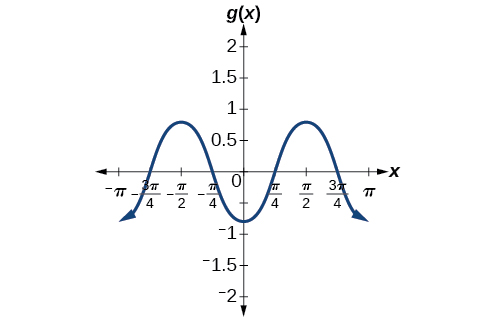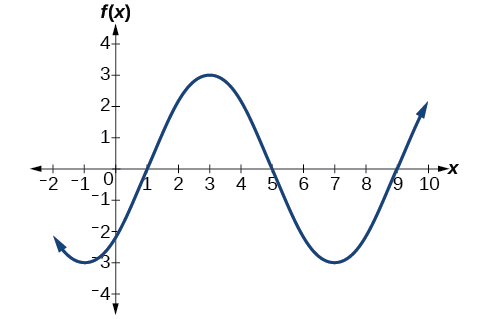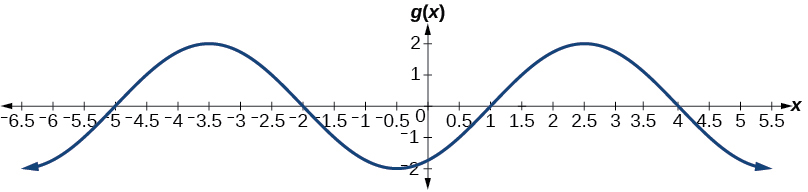# 8.1 Graphs of the sine and cosine functions  (Page 5/13)

 Page 5 / 13

Write a formula for the function graphed in [link] .

two possibilities: $\text{\hspace{0.17em}}y=4\mathrm{sin}\left(\frac{\pi }{5}x-\frac{\pi }{5}\right)+4\text{\hspace{0.17em}}$ or $\text{\hspace{0.17em}}y=-4\mathrm{sin}\left(\frac{\pi }{5}x+\frac{4\pi }{5}\right)+4$

## Graphing variations of y = sin x And y = cos x

Throughout this section, we have learned about types of variations of sine and cosine functions and used that information to write equations from graphs. Now we can use the same information to create graphs from equations.

Instead of focusing on the general form equations

we will let $\text{\hspace{0.17em}}C=0\text{\hspace{0.17em}}$ and $\text{\hspace{0.17em}}D=0\text{\hspace{0.17em}}$ and work with a simplified form of the equations in the following examples.

Given the function $\text{\hspace{0.17em}}y=A\mathrm{sin}\left(Bx\right),\text{\hspace{0.17em}}$ sketch its graph.

1. Identify the amplitude, $\text{\hspace{0.17em}}|A|.$
2. Identify the period, $\text{\hspace{0.17em}}P=\frac{2\pi }{|B|}.$
3. Start at the origin, with the function increasing to the right if $\text{\hspace{0.17em}}A\text{\hspace{0.17em}}$ is positive or decreasing if $\text{\hspace{0.17em}}A\text{\hspace{0.17em}}$ is negative.
4. At $\text{\hspace{0.17em}}x=\frac{\pi }{2|B|}\text{\hspace{0.17em}}$ there is a local maximum for $\text{\hspace{0.17em}}A>0\text{\hspace{0.17em}}$ or a minimum for $\text{\hspace{0.17em}}A<0,\text{\hspace{0.17em}}$ with $\text{\hspace{0.17em}}y=A.$
5. The curve returns to the x -axis at $\text{\hspace{0.17em}}x=\frac{\pi }{|B|}.$
6. There is a local minimum for $\text{\hspace{0.17em}}A>0\text{\hspace{0.17em}}$ (maximum for $\text{\hspace{0.17em}}A<0$ ) at $\text{\hspace{0.17em}}x=\frac{3\pi }{2|B|}\text{\hspace{0.17em}}$ with $\text{\hspace{0.17em}}y=–A.$
7. The curve returns again to the x -axis at $\text{\hspace{0.17em}}x=\frac{\pi }{2|B|}.$

## Graphing a function and identifying the amplitude and period

Sketch a graph of $\text{\hspace{0.17em}}f\left(x\right)=-2\mathrm{sin}\left(\frac{\pi x}{2}\right).$

Let’s begin by comparing the equation to the form $\text{\hspace{0.17em}}y=A\mathrm{sin}\left(Bx\right).$

• Step 1. We can see from the equation that $\text{\hspace{0.17em}}A=-2,$ so the amplitude is 2.
$|A|=2$
• Step 2. The equation shows that $\text{\hspace{0.17em}}B=\frac{\pi }{2},\text{\hspace{0.17em}}$ so the period is
• Step 3. Because $\text{\hspace{0.17em}}A\text{\hspace{0.17em}}$ is negative, the graph descends as we move to the right of the origin.
• Step 4–7. The x -intercepts are at the beginning of one period, $\text{\hspace{0.17em}}x=0,\text{\hspace{0.17em}}$ the horizontal midpoints are at $\text{\hspace{0.17em}}x=2\text{\hspace{0.17em}}$ and at the end of one period at $\text{\hspace{0.17em}}x=4.$

The quarter points include the minimum at $\text{\hspace{0.17em}}x=1\text{\hspace{0.17em}}$ and the maximum at $\text{\hspace{0.17em}}x=3.\text{\hspace{0.17em}}$ A local minimum will occur 2 units below the midline, at $\text{\hspace{0.17em}}x=1,\text{\hspace{0.17em}}$ and a local maximum will occur at 2 units above the midline, at $\text{\hspace{0.17em}}x=3.\text{\hspace{0.17em}}$ [link] shows the graph of the function.

Sketch a graph of $\text{\hspace{0.17em}}g\left(x\right)=-0.8\mathrm{cos}\left(2x\right).\text{\hspace{0.17em}}$ Determine the midline, amplitude, period, and phase shift.midline: $\text{\hspace{0.17em}}y=0;\text{\hspace{0.17em}}$ amplitude: $\text{\hspace{0.17em}}|A|=0.8;\text{\hspace{0.17em}}$ period: $\text{\hspace{0.17em}}P=\frac{2\pi }{|B|}=\pi ;\text{\hspace{0.17em}}$ phase shift: $\text{\hspace{0.17em}}\frac{C}{B}=0\text{\hspace{0.17em}}$ or none

Given a sinusoidal function with a phase shift and a vertical shift, sketch its graph.

1. Express the function in the general form
2. Identify the amplitude, $\text{\hspace{0.17em}}|A|.$
3. Identify the period, $\text{\hspace{0.17em}}P=\frac{2\pi }{|B|}.$
4. Identify the phase shift, $\text{\hspace{0.17em}}\frac{C}{B}.$
5. Draw the graph of $\text{\hspace{0.17em}}f\left(x\right)=A\mathrm{sin}\left(Bx\right)\text{\hspace{0.17em}}$ shifted to the right or left by $\text{\hspace{0.17em}}\frac{C}{B}\text{\hspace{0.17em}}$ and up or down by $\text{\hspace{0.17em}}D.$

## Graphing a transformed sinusoid

Sketch a graph of $\text{\hspace{0.17em}}f\left(x\right)=3\mathrm{sin}\left(\frac{\pi }{4}x-\frac{\pi }{4}\right).$

• Step 1. The function is already written in general form: $\text{\hspace{0.17em}}f\left(x\right)=3\mathrm{sin}\left(\frac{\pi }{4}x-\frac{\pi }{4}\right).$ This graph will have the shape of a sine function    , starting at the midline and increasing to the right.
• Step 2. $\text{\hspace{0.17em}}|A|=|3|=3.\text{\hspace{0.17em}}$ The amplitude is 3.
• Step 3. Since $\text{\hspace{0.17em}}|B|=|\frac{\pi }{4}|=\frac{\pi }{4},\text{\hspace{0.17em}}$ we determine the period as follows.
$P=\frac{2\pi }{|B|}=\frac{2\pi }{\frac{\pi }{4}}=2\pi \cdot \frac{4}{\pi }=8$

The period is 8.

• Step 4. Since $\text{\hspace{0.17em}}C=\frac{\pi }{4},\text{\hspace{0.17em}}$ the phase shift is
$\frac{C}{B}=\frac{\frac{\pi }{4}}{\frac{\pi }{4}}=1.$

The phase shift is 1 unit.

• Step 5. [link] shows the graph of the function.A horizontally compressed, vertically stretched, and horizontally shifted sinusoid

Draw a graph of $\text{\hspace{0.17em}}g\left(x\right)=-2\mathrm{cos}\left(\frac{\pi }{3}x+\frac{\pi }{6}\right).\text{\hspace{0.17em}}$ Determine the midline, amplitude, period, and phase shift.midline: $\text{\hspace{0.17em}}y=0;\text{\hspace{0.17em}}$ amplitude: $\text{\hspace{0.17em}}|A|=2;\text{\hspace{0.17em}}$ period: $\text{\hspace{0.17em}}P=\frac{2\pi }{|B|}=6;\text{\hspace{0.17em}}$ phase shift: $\text{\hspace{0.17em}}\frac{C}{B}=-\frac{1}{2}$

#### Questions & Answers

A laser rangefinder is locked on a comet approaching Earth. The distance g(x), in kilometers, of the comet after x days, for x in the interval 0 to 30 days, is given by g(x)=250,000csc(π30x). Graph g(x) on the interval [0, 35]. Evaluate g(5)  and interpret the information. What is the minimum distance between the comet and Earth? When does this occur? To which constant in the equation does this correspond? Find and discuss the meaning of any vertical asymptotes.
Kaitlyn Reply
The sequence is {1,-1,1-1.....} has
amit Reply
circular region of radious
Kainat Reply
how can we solve this problem
Joel Reply
Sin(A+B) = sinBcosA+cosBsinA
Eseka Reply
Prove it
Eseka
Please prove it
Eseka
hi
Joel
June needs 45 gallons of punch. 2 different coolers. Bigger cooler is 5 times as large as smaller cooler. How many gallons in each cooler?
Arleathia Reply
7.5 and 37.5
Nando
find the sum of 28th term of the AP 3+10+17+---------
Prince Reply
I think you should say "28 terms" instead of "28th term"
Vedant
the 28th term is 175
Nando
192
Kenneth
if sequence sn is a such that sn>0 for all n and lim sn=0than prove that lim (s1 s2............ sn) ke hole power n =n
SANDESH Reply
write down the polynomial function with root 1/3,2,-3 with solution
Gift Reply
if A and B are subspaces of V prove that (A+B)/B=A/(A-B)
Pream Reply
write down the value of each of the following in surd form a)cos(-65°) b)sin(-180°)c)tan(225°)d)tan(135°)
Oroke Reply
Prove that (sinA/1-cosA - 1-cosA/sinA) (cosA/1-sinA - 1-sinA/cosA) = 4
kiruba Reply
what is the answer to dividing negative index
Morosi Reply
In a triangle ABC prove that. (b+c)cosA+(c+a)cosB+(a+b)cisC=a+b+c.
Shivam Reply
give me the waec 2019 questions
Aaron Reply

### Read also:

#### Get the best Algebra and trigonometry course in your pocket!

Source:  OpenStax, Algebra and trigonometry. OpenStax CNX. Nov 14, 2016 Download for free at https://legacy.cnx.org/content/col11758/1.6
Google Play and the Google Play logo are trademarks of Google Inc.

Notification Switch

Would you like to follow the 'Algebra and trigonometry' conversation and receive update notifications?ByByByBy RhodesBy